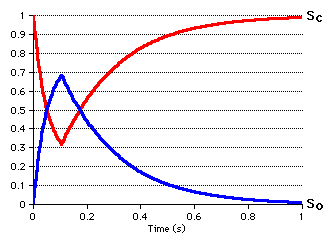# Markov

### Introduction

This program demonstrates the use of the Markov class. A simple two-state Markov model is created. In anticipation of future use as a Markov synapse, we'll call the two states "Closed" (Sc) and "Open" (So).

16.0
.-------> So
Sc <-------'
4.7

The model starts out 100% closed (i.e., Sc=1 and Sc=0 when t=0). As the simulation begins, both forward and reverse transitions are permitted, resulting in an exponential drop (see below). At t=0.1, the forward flow (i.e., Sc ----> So ) is blocked. Conceptually, we are simulating a forward rate which requires a ligand only present between t=0 and t=0.1. After this time, the reverse ( Sc <---- So ) flow dominates, and the model returns completely to the closed state.

### Code Overview

The complete code for the program is contained in main.cpp. It is fairly short, and can be summarized as follows.
1. Create the Markov model and set its transition rates.
2. Bind the forward rate to a "Ligand concentration" variable.
3. Loop through time. At each time step,
1. Output variables of interest
2. Call the model's Step() method (inherited from Stepper)
3. Update the ligand concentration (when appropriate)
In step 3.2, we could have used gStepper.StepAll() instead; this is the generally preferred method. But in the case of an isolated Markov model, it works to call its Step() method directly.

Note that in the code, C and P are used instead of Sc and So to refer to the states.

### Program Output

The actual output of this program is a table of numbers, which has been graphed (by importing the data to ClarisWorks) below. Note that the sum of the states always equals one (Sc + So == 1).### Building the Program

If you have the full set of CONICAL source code, you should be able to simply copy main.cpp into your project and build it. Of course, this will contain quite a few files you don't need. A minimal project would contain just the following files:

Source FilesHeader Files
main.cpp
Marxov.cpp
Stepmstr.cpp
Markov.h
Stepper.h
Stepmstr.h

(Notice that the Stepmaster code is required when any Stepper is used, even if the Stepmaster's methods are not explicitly called.)